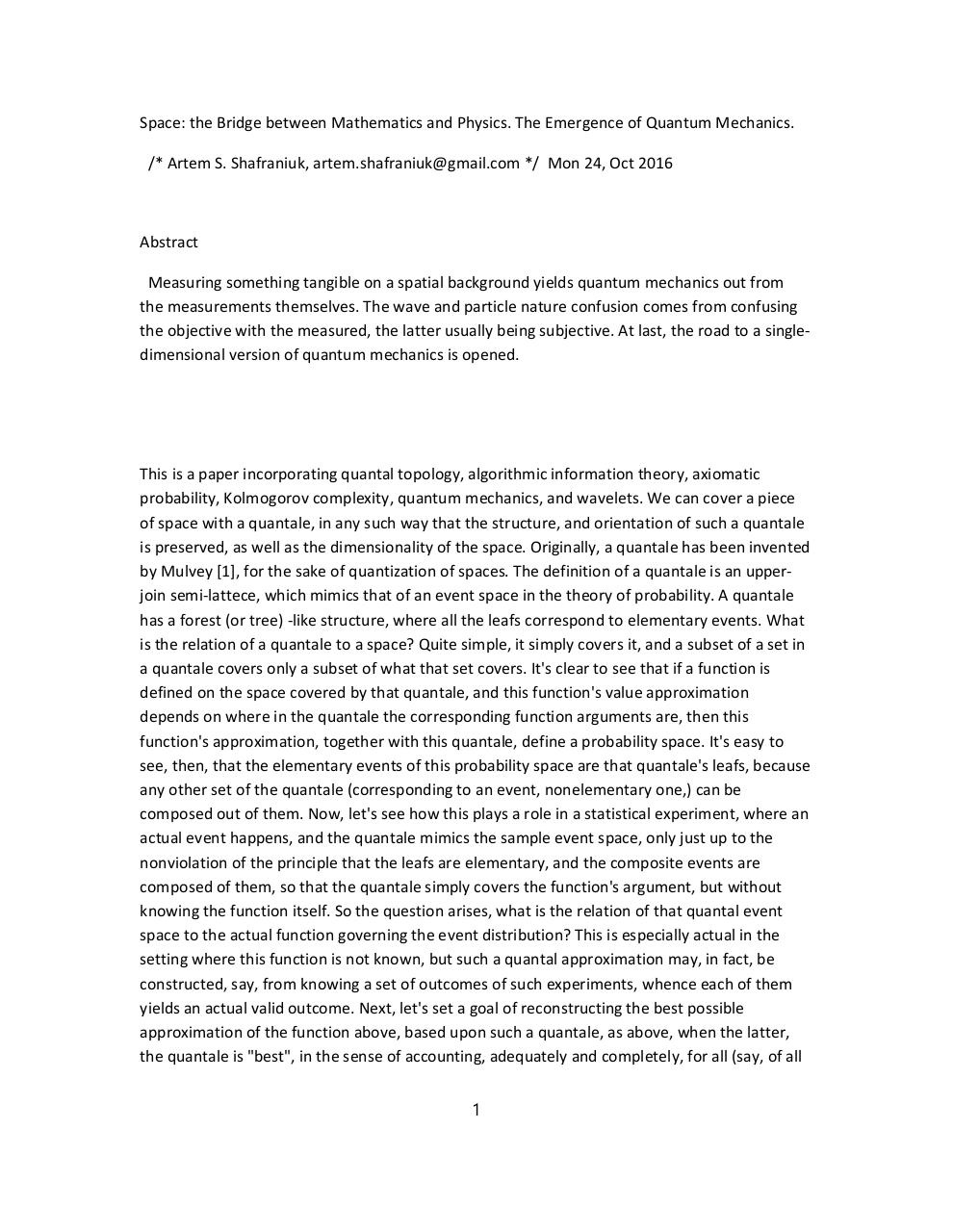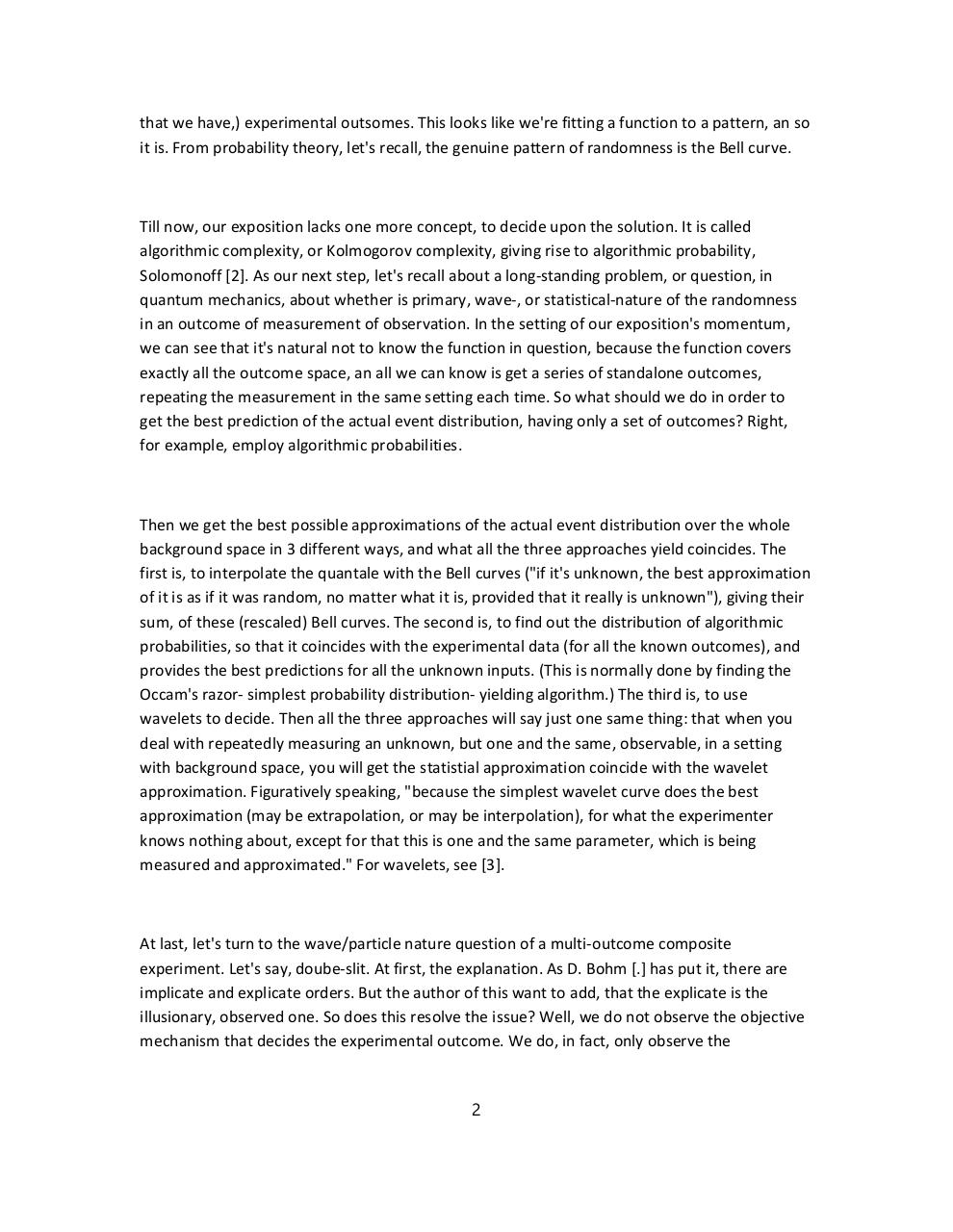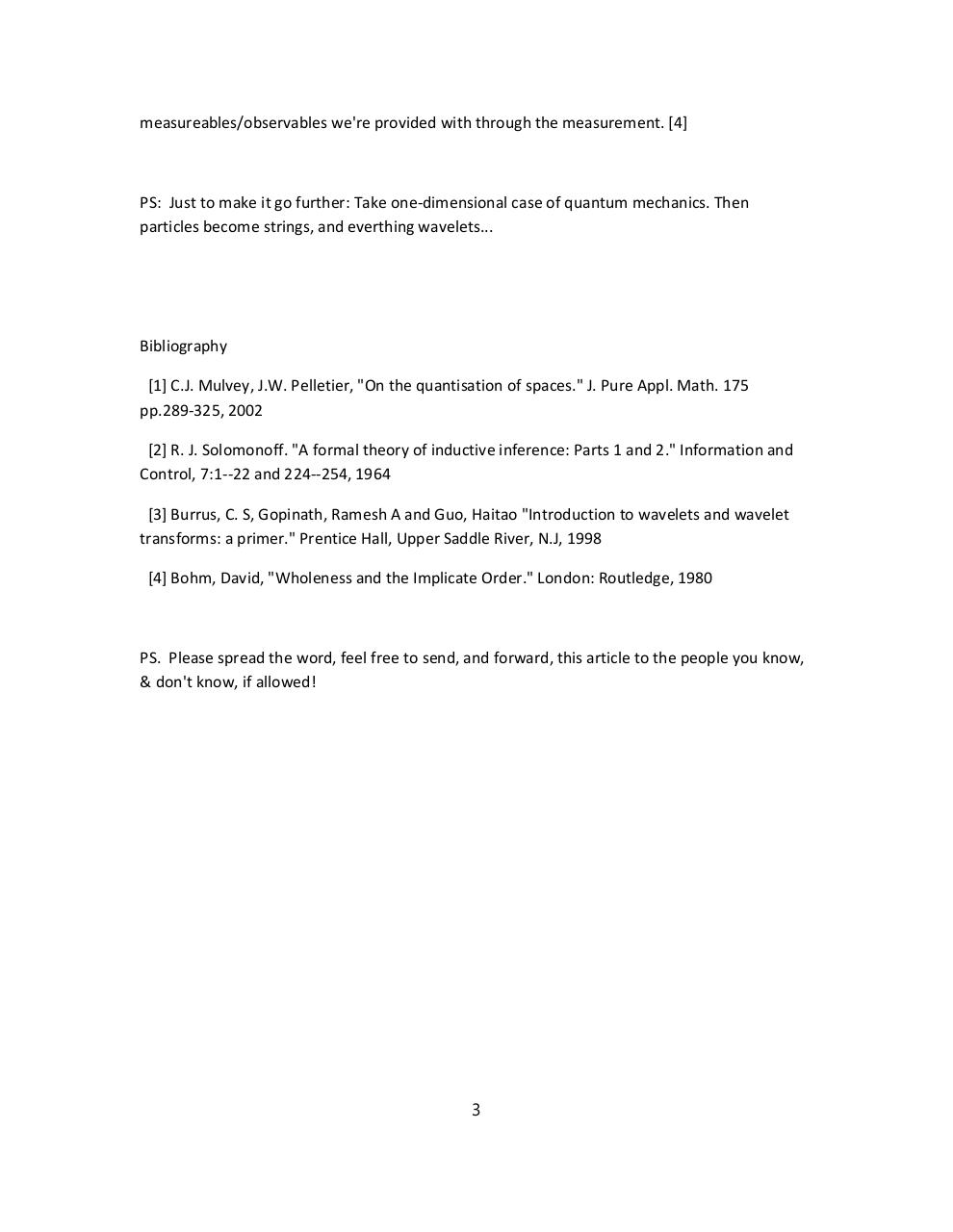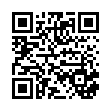# quantalm .pdf

### File information

Original filename: quantalm.pdf
Title: quantalm.rtf
Author: artem

This PDF 1.7 document has been generated by / Microsoft: Print To PDF, and has been sent on pdf-archive.com on 25/10/2016 at 22:51, from IP address 107.135.x.x. The current document download page has been viewed 410 times.
File size: 214 KB (3 pages).
Privacy: public file

quantalm.pdf (PDF, 214 KB)

### Document preview

Space: the Bridge between Mathematics and Physics. The Emergence of Quantum Mechanics.
/* Artem S. Shafraniuk, artem.shafraniuk@gmail.com */ Mon 24, Oct 2016

Abstract

Measuring something tangible on a spatial background yields quantum mechanics out from
the measurements themselves. The wave and particle nature confusion comes from confusing
the objective with the measured, the latter usually being subjective. At last, the road to a singledimensional version of quantum mechanics is opened.

This is a paper incorporating quantal topology, algorithmic information theory, axiomatic
probability, Kolmogorov complexity, quantum mechanics, and wavelets. We can cover a piece
of space with a quantale, in any such way that the structure, and orientation of such a quantale
is preserved, as well as the dimensionality of the space. Originally, a quantale has been invented
by Mulvey , for the sake of quantization of spaces. The definition of a quantale is an upperjoin semi-lattece, which mimics that of an event space in the theory of probability. A quantale
has a forest (or tree) -like structure, where all the leafs correspond to elementary events. What
is the relation of a quantale to a space? Quite simple, it simply covers it, and a subset of a set in
a quantale covers only a subset of what that set covers. It's clear to see that if a function is
defined on the space covered by that quantale, and this function's value approximation
depends on where in the quantale the corresponding function arguments are, then this
function's approximation, together with this quantale, define a probability space. It's easy to
see, then, that the elementary events of this probability space are that quantale's leafs, because
any other set of the quantale (corresponding to an event, nonelementary one,) can be
composed out of them. Now, let's see how this plays a role in a statistical experiment, where an
actual event happens, and the quantale mimics the sample event space, only just up to the
nonviolation of the principle that the leafs are elementary, and the composite events are
composed of them, so that the quantale simply covers the function's argument, but without
knowing the function itself. So the question arises, what is the relation of that quantal event
space to the actual function governing the event distribution? This is especially actual in the
setting where this function is not known, but such a quantal approximation may, in fact, be
constructed, say, from knowing a set of outcomes of such experiments, whence each of them
yields an actual valid outcome. Next, let's set a goal of reconstructing the best possible
approximation of the function above, based upon such a quantale, as above, when the latter,
the quantale is "best", in the sense of accounting, adequately and completely, for all (say, of all
1

that we have,) experimental outsomes. This looks like we're fitting a function to a pattern, an so
it is. From probability theory, let's recall, the genuine pattern of randomness is the Bell curve.
Till now, our exposition lacks one more concept, to decide upon the solution. It is called
algorithmic complexity, or Kolmogorov complexity, giving rise to algorithmic probability,
Solomonoff . As our next step, let's recall about a long-standing problem, or question, in
quantum mechanics, about whether is primary, wave-, or statistical-nature of the randomness
in an outcome of measurement of observation. In the setting of our exposition's momentum,
we can see that it's natural not to know the function in question, because the function covers
exactly all the outcome space, an all we can know is get a series of standalone outcomes,
repeating the measurement in the same setting each time. So what should we do in order to
for example, employ algorithmic probabilities.
background space in 3 different ways, and what all the three approaches yield coincides. The
first is, to interpolate the quantale with the Bell curves ("if it's unknown, the best approximation
of it is as if it was random, no matter what it is, provided that it really is unknown"), giving their
sum, of these (rescaled) Bell curves. The second is, to find out the distribution of algorithmic
probabilities, so that it coincides with the experimental data (for all the known outcomes), and
provides the best predictions for all the unknown inputs. (This is normally done by finding the
Occam's razor- simplest probability distribution- yielding algorithm.) The third is, to use
wavelets to decide. Then all the three approaches will say just one same thing: that when you
deal with repeatedly measuring an unknown, but one and the same, observable, in a setting
with background space, you will get the statistial approximation coincide with the wavelet
approximation. Figuratively speaking, "because the simplest wavelet curve does the best
approximation (may be extrapolation, or may be interpolation), for what the experimenter
knows nothing about, except for that this is one and the same parameter, which is being
measured and approximated." For wavelets, see .
At last, let's turn to the wave/particle nature question of a multi-outcome composite
experiment. Let's say, doube-slit. At first, the explanation. As D. Bohm [.] has put it, there are
implicate and explicate orders. But the author of this want to add, that the explicate is the
illusionary, observed one. So does this resolve the issue? Well, we do not observe the objective
mechanism that decides the experimental outcome. We do, in fact, only observe the
2

measureables/observables we're provided with through the measurement. 
PS: Just to make it go further: Take one-dimensional case of quantum mechanics. Then
particles become strings, and everthing wavelets...

Bibliography

 C.J. Mulvey, J.W. Pelletier, "On the quantisation of spaces." J. Pure Appl. Math. 175
pp.289-325, 2002

 R. J. Solomonoff. "A formal theory of inductive inference: Parts 1 and 2." Information and
Control, 7:1--22 and 224--254, 1964
 Burrus, C. S, Gopinath, Ramesh A and Guo, Haitao "Introduction to wavelets and wavelet
transforms: a primer." Prentice Hall, Upper Saddle River, N.J, 1998
 Bohm, David, "Wholeness and the Implicate Order." London: Routledge, 1980

&amp; don't know, if allowed!

3#### HTML Code

Copy the following HTML code to share your document on a Website or Blog

#### QR Code### Related keywords